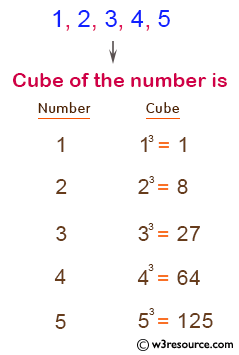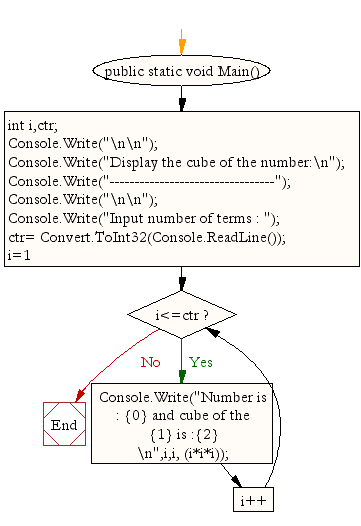﻿ C# - Find cube of the number up to given an integer# C# Sharp Exercises: Find cube of the number up to given an integer

## C# Sharp For Loop: Exercise-5 with Solution

Write a program in C# Sharp to display the cube of the number up to given an integer.

Pictorial Presentation:Sample Solution:

C# Sharp Code:

``````using System;
public class Exercise5
{
public static void Main()
{
int i,ctr;
Console.Write("\n\n");
Console.Write("Display the cube of the number:\n");
Console.Write("---------------------------------");
Console.Write("\n\n");

Console.Write("Input number of terms : ");
for(i=1;i<=ctr;i++)
{
Console.Write("Number is : {0} and cube of the {1} is :{2} \n",i,i, (i*i*i));
}
}
}
```
```

Sample Output:

```Display the cube of the number:
---------------------------------

Input number of terms : 5
Number is : 1 and cube of the 1 is :1
Number is : 2 and cube of the 2 is :8
Number is : 3 and cube of the 3 is :27
Number is : 4 and cube of the 4 is :64
Number is : 5 and cube of the 5 is :125
```

Flowchart:C# Sharp Code Editor:

Contribute your code and comments through Disqus.

What is the difficulty level of this exercise?

Test your Programming skills with w3resource's quiz.

﻿